##### The Japanese Bonsai specialist
Direct order Contact Help / Services Newsletter# Hakonechloa all gold japanese forest grass

Hakonecloa macra "all gold"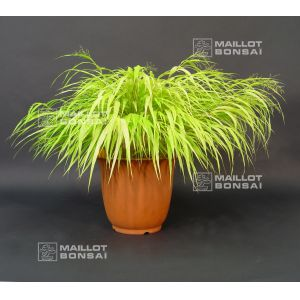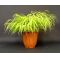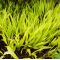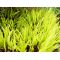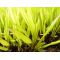ref. : 6912

7,00

Available quantity : 3Order

###### Description

Japanese grass, vivacious plant, short creeping rhizomes. Delivered as a tuft of grass in a 1 litre plastic pot. Hakonechloa All Gold adds a burst of colour to Japanese garden flowerbeds and sunny balconies and patios. Its leaves are green in May and turn golden yellow at the end of September. In autumn they're a red colour, transforming to brown in winter. This plant is easy to grow with regular watering and it flowers at the end of summer.

#japanese 4.8 #grass 4.5 #hakonechloa 4.1 #gold 3.4 #kusamono 2.8 #forest 2.6 #colour 2.6 #plants 2.6 #plant 2.5 #small 2.5

Formule
(( ROUND((CHAR_LENGTH(b.article_nom)-CHAR_LENGTH(REPLACE(b.article_nom, 'japanese', '')))/LENGTH('japanese')) + ROUND((CHAR_LENGTH(b.article_description)-CHAR_LENGTH(REPLACE(b.article_description, 'japanese', '')))/LENGTH('japanese')) ) * 4.8) + (( ROUND((CHAR_LENGTH(b.article_nom)-CHAR_LENGTH(REPLACE(b.article_nom, 'grass', '')))/LENGTH('grass')) + ROUND((CHAR_LENGTH(b.article_description)-CHAR_LENGTH(REPLACE(b.article_description, 'grass', '')))/LENGTH('grass')) ) * 4.5) + (( ROUND((CHAR_LENGTH(b.article_nom)-CHAR_LENGTH(REPLACE(b.article_nom, 'hakonechloa', '')))/LENGTH('hakonechloa')) + ROUND((CHAR_LENGTH(b.article_description)-CHAR_LENGTH(REPLACE(b.article_description, 'hakonechloa', '')))/LENGTH('hakonechloa')) ) * 4.1) + (( ROUND((CHAR_LENGTH(b.article_nom)-CHAR_LENGTH(REPLACE(b.article_nom, 'gold', '')))/LENGTH('gold')) + ROUND((CHAR_LENGTH(b.article_description)-CHAR_LENGTH(REPLACE(b.article_description, 'gold', '')))/LENGTH('gold')) ) * 3.4) + (( ROUND((CHAR_LENGTH(b.article_nom)-CHAR_LENGTH(REPLACE(b.article_nom, 'forest', '')))/LENGTH('forest')) + ROUND((CHAR_LENGTH(b.article_description)-CHAR_LENGTH(REPLACE(b.article_description, 'forest', '')))/LENGTH('forest')) ) * 2.6) + (( ROUND((CHAR_LENGTH(b.article_nom)-CHAR_LENGTH(REPLACE(b.article_nom, 'colour', '')))/LENGTH('colour')) + ROUND((CHAR_LENGTH(b.article_description)-CHAR_LENGTH(REPLACE(b.article_description, 'colour', '')))/LENGTH('colour')) ) * 2.6) + (( ROUND((CHAR_LENGTH(b.article_nom)-CHAR_LENGTH(REPLACE(b.article_nom, 'plant', '')))/LENGTH('plant')) + ROUND((CHAR_LENGTH(b.article_description)-CHAR_LENGTH(REPLACE(b.article_description, 'plant', '')))/LENGTH('plant')) ) * 2.5) + (( ROUND((CHAR_LENGTH(b.article_nom)-CHAR_LENGTH(REPLACE(b.article_nom, 'transforming', '')))/LENGTH('transforming')) + ROUND((CHAR_LENGTH(b.article_description)-CHAR_LENGTH(REPLACE(b.article_description, 'transforming', '')))/LENGTH('transforming')) ) * 2.2) + (( ROUND((CHAR_LENGTH(b.article_nom)-CHAR_LENGTH(REPLACE(b.article_nom, 'flowerbeds', '')))/LENGTH('flowerbeds')) + ROUND((CHAR_LENGTH(b.article_description)-CHAR_LENGTH(REPLACE(b.article_description, 'flowerbeds', '')))/LENGTH('flowerbeds')) ) * 2) + (( ROUND((CHAR_LENGTH(b.article_nom)-CHAR_LENGTH(REPLACE(b.article_nom, 'vivacious', '')))/LENGTH('vivacious')) + ROUND((CHAR_LENGTH(b.article_description)-CHAR_LENGTH(REPLACE(b.article_description, 'vivacious', '')))/LENGTH('vivacious')) ) * 1.9)

## Secure payment## Delivery

Our logistic partners :04 74 55 23 48
Pépinière MAILLOT-BONSAÏ
Le Bois Frazy
01990 RELEVANT - FRANCE
on appointmentThe Japanese Bonsai specialist
If you like Maillot Bonsaï, share on  facebook

A vos côtés. Les services restent les mêmes.

La vente continue et les expéditions également.

Meilleurs vœux pour 2021.

Guy Maillot

OK